10. Can the outline of a Tetrad form a regular polygon?

Yes: This seems to be possible for all polygons of order n, except when n = 4.

n = 3   Triangle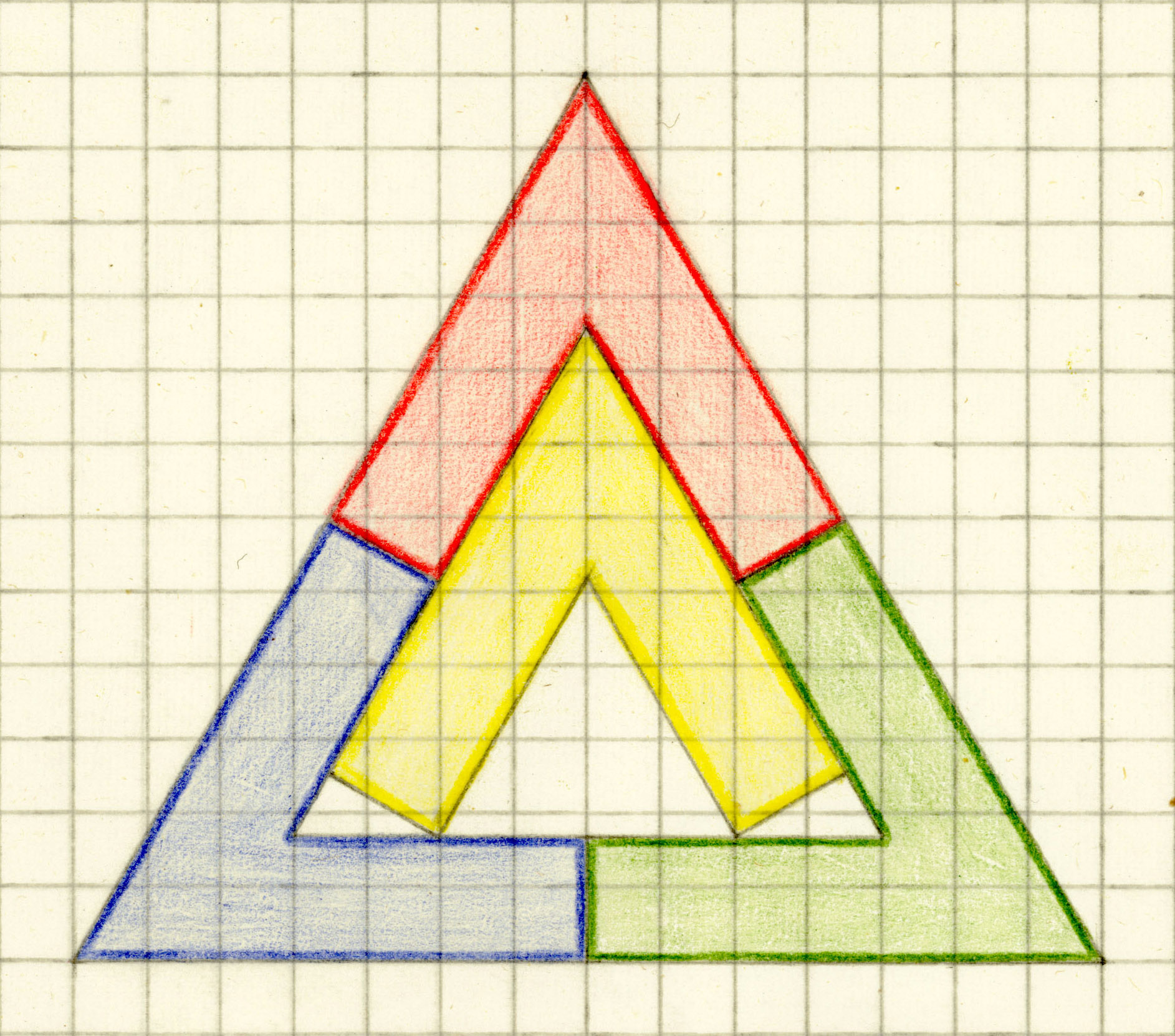Open problem: Can triangles which are not equilateral be the outer boundary of a tetrad?

n = 4   Square

The picture just shows that it is impossible to create a square tetrad where the outline is formed by only two tiles. The sum of the two short lines is smaller than 1. A complete proof is not given here.The problem is easy to solve for rectangles with width < length < 2 · width and for parallelograms.

 Open problem: Which trapezoids or kites can be the outer boundary of a tetrad?

n = 5   Pentagon

The next figure shows a possible construction principle and an incomplete solution, where the inner tile and the blue tile have only one point in common.The problem can be solved. In the second solution the inner tile (yellow) has left its original position.Another challenge is to minimize the area of the holes.n = 6   Hexagon
This can be done with hexagon tiles. the first tetrad and its tiles are mirror symmetric.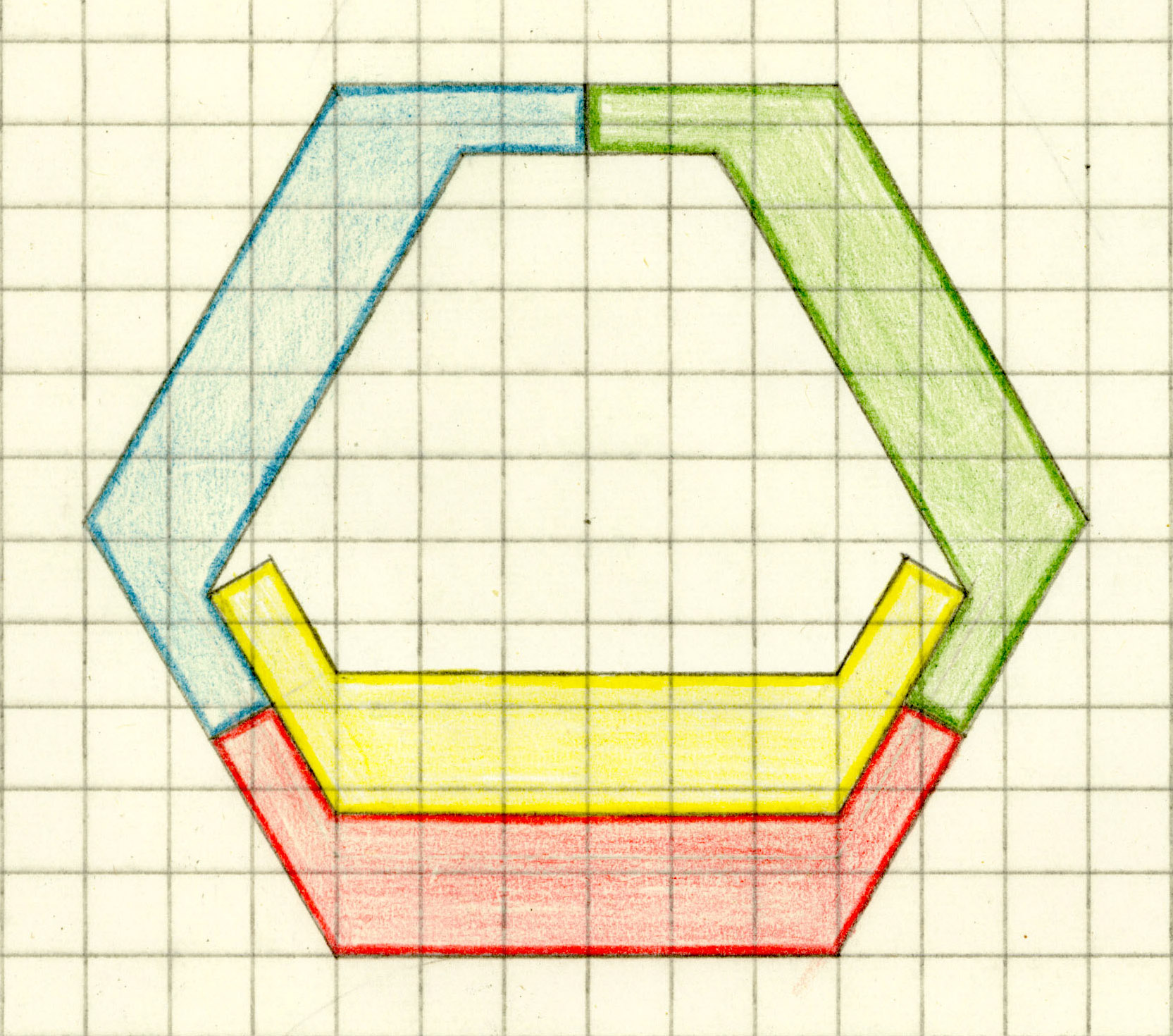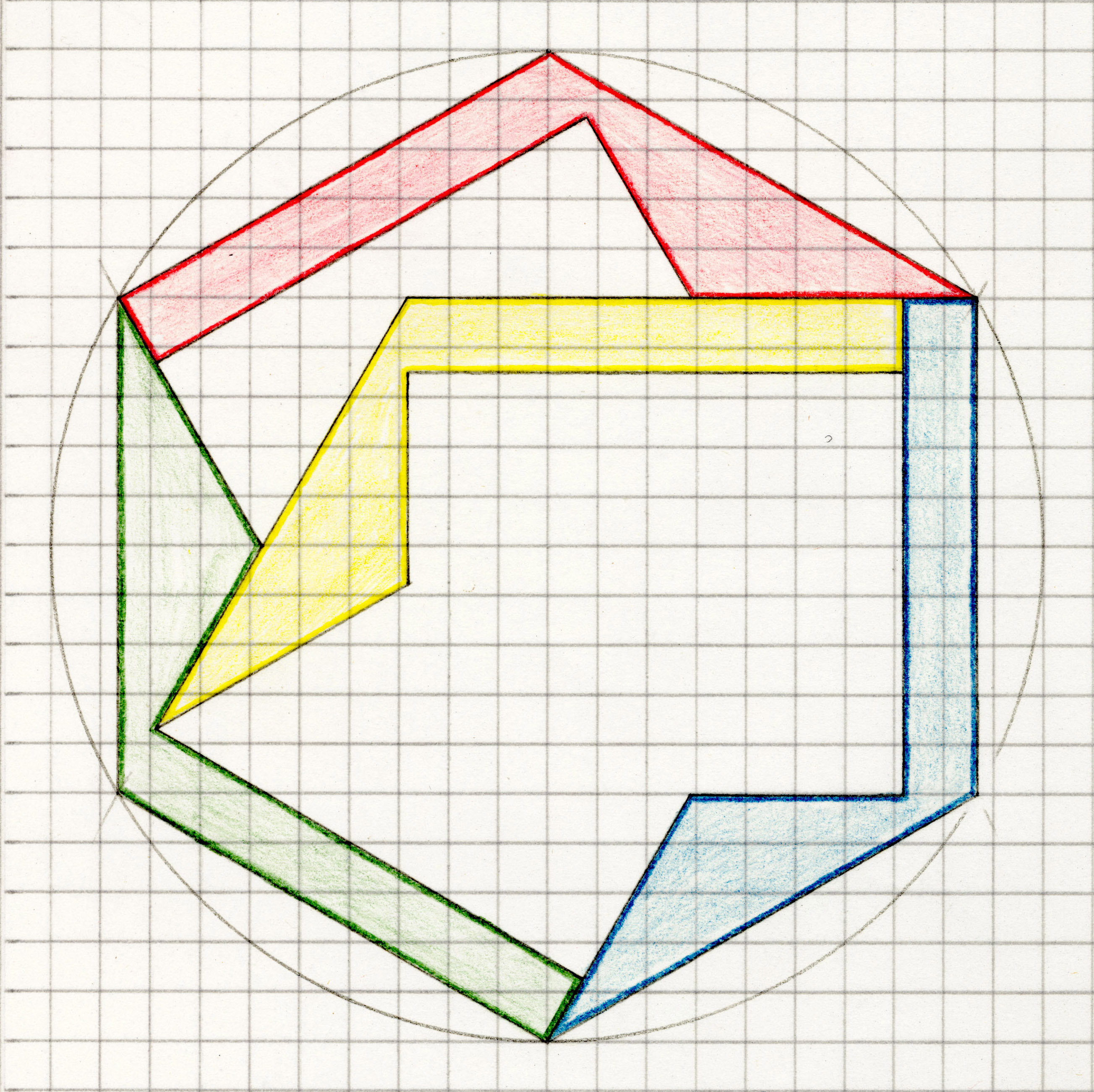You also can use heptagon tiles.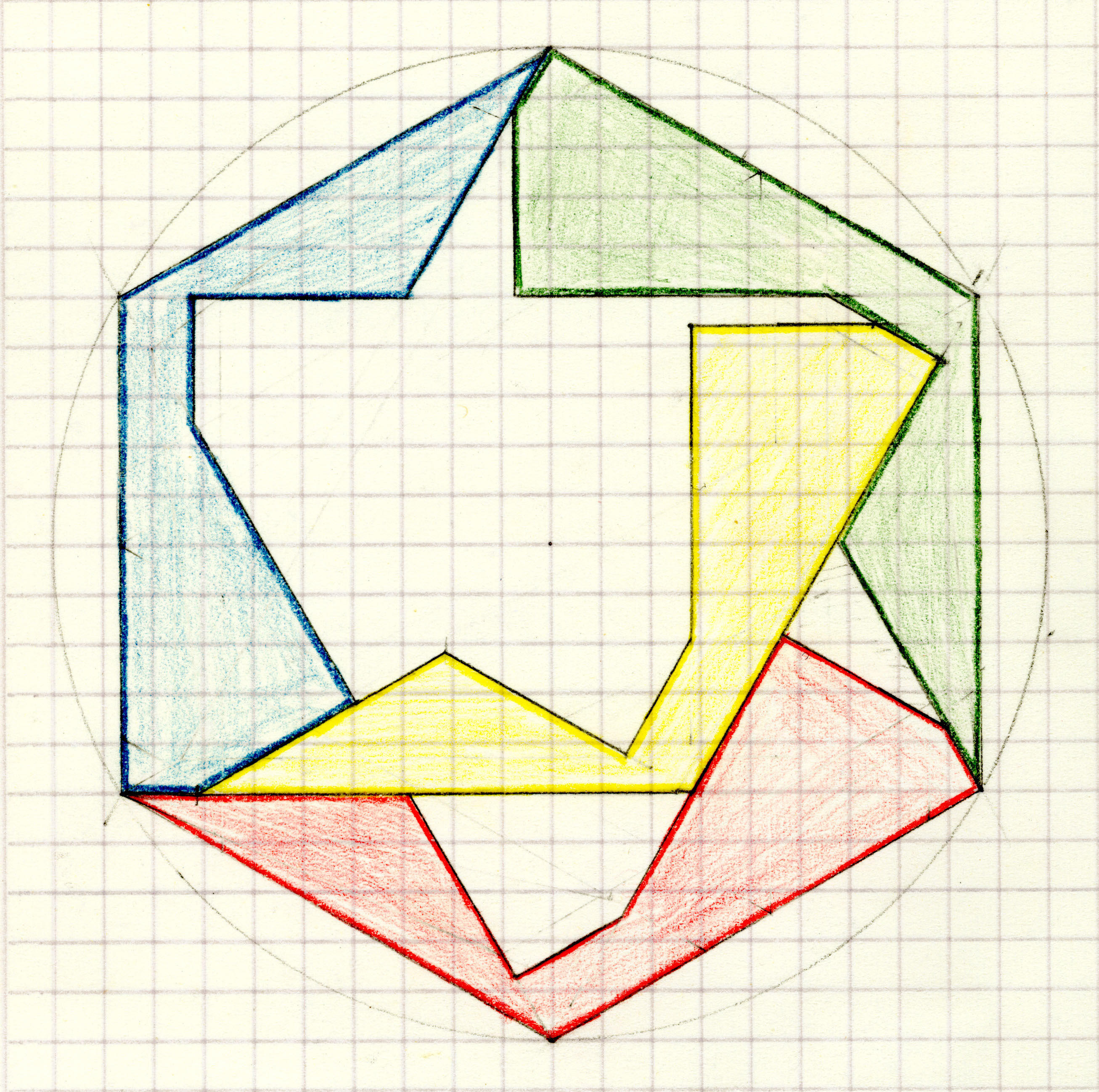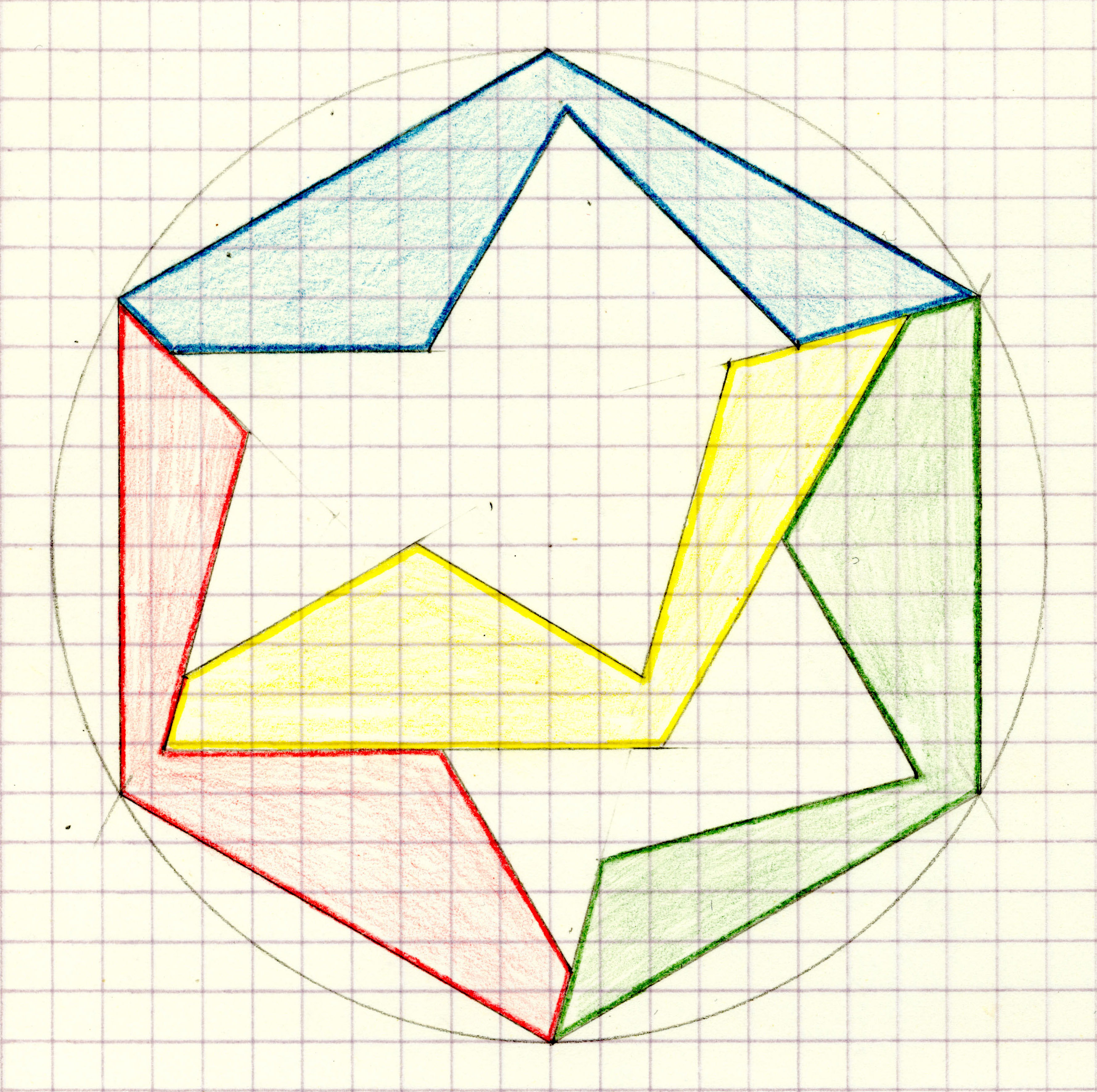n = 7   Heptagon     Should be proved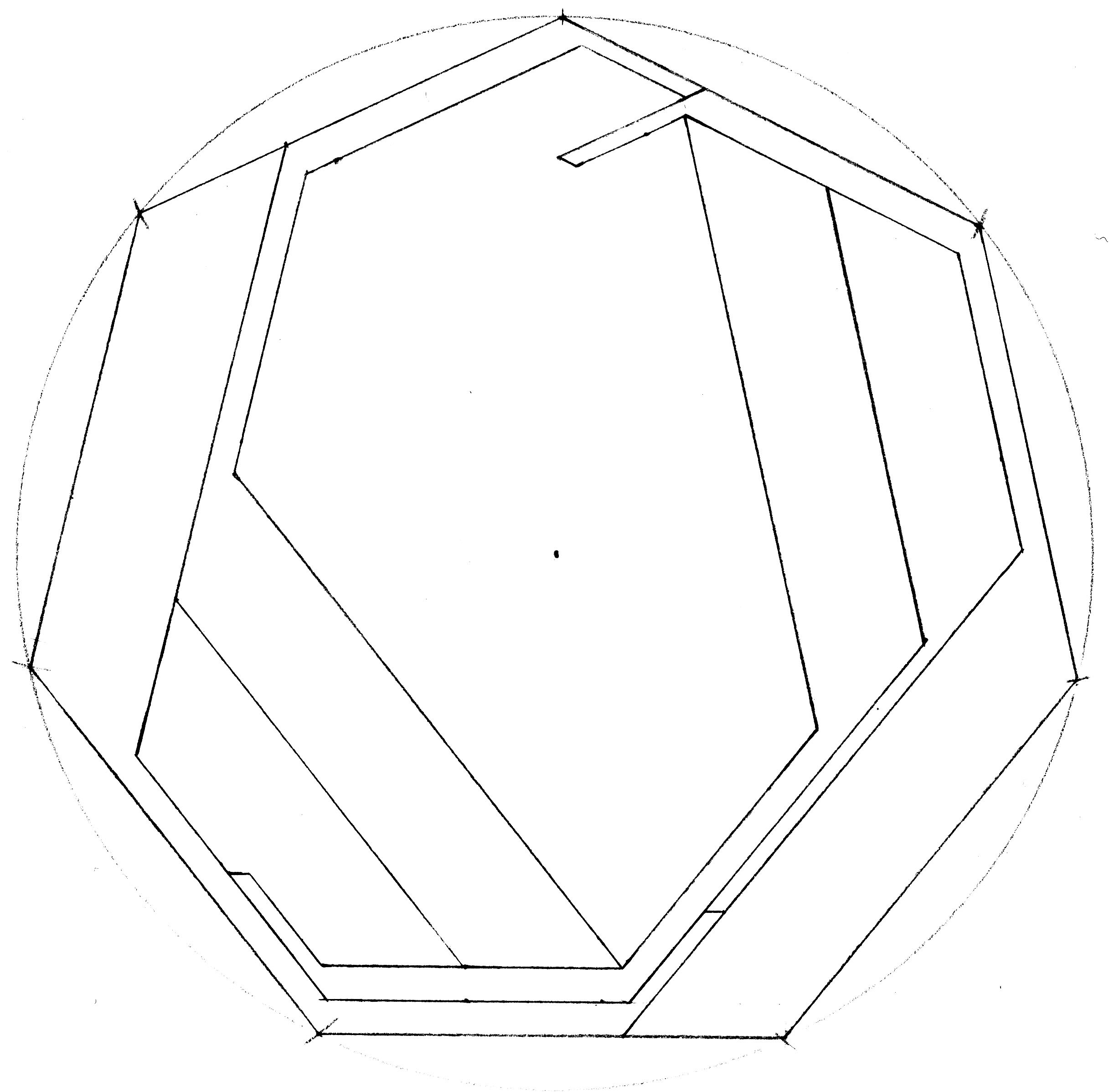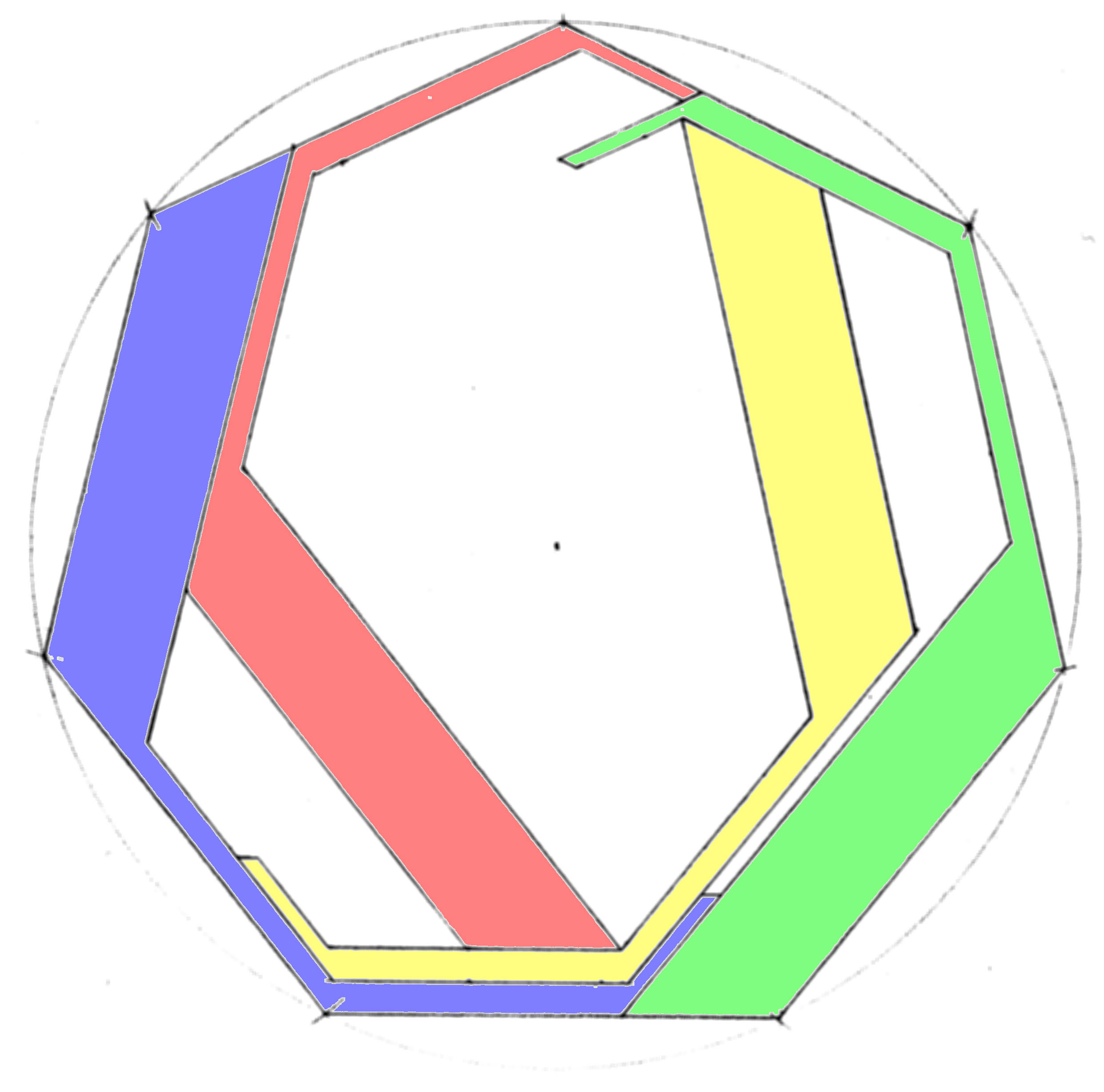n = 8   Octagon     Should be proved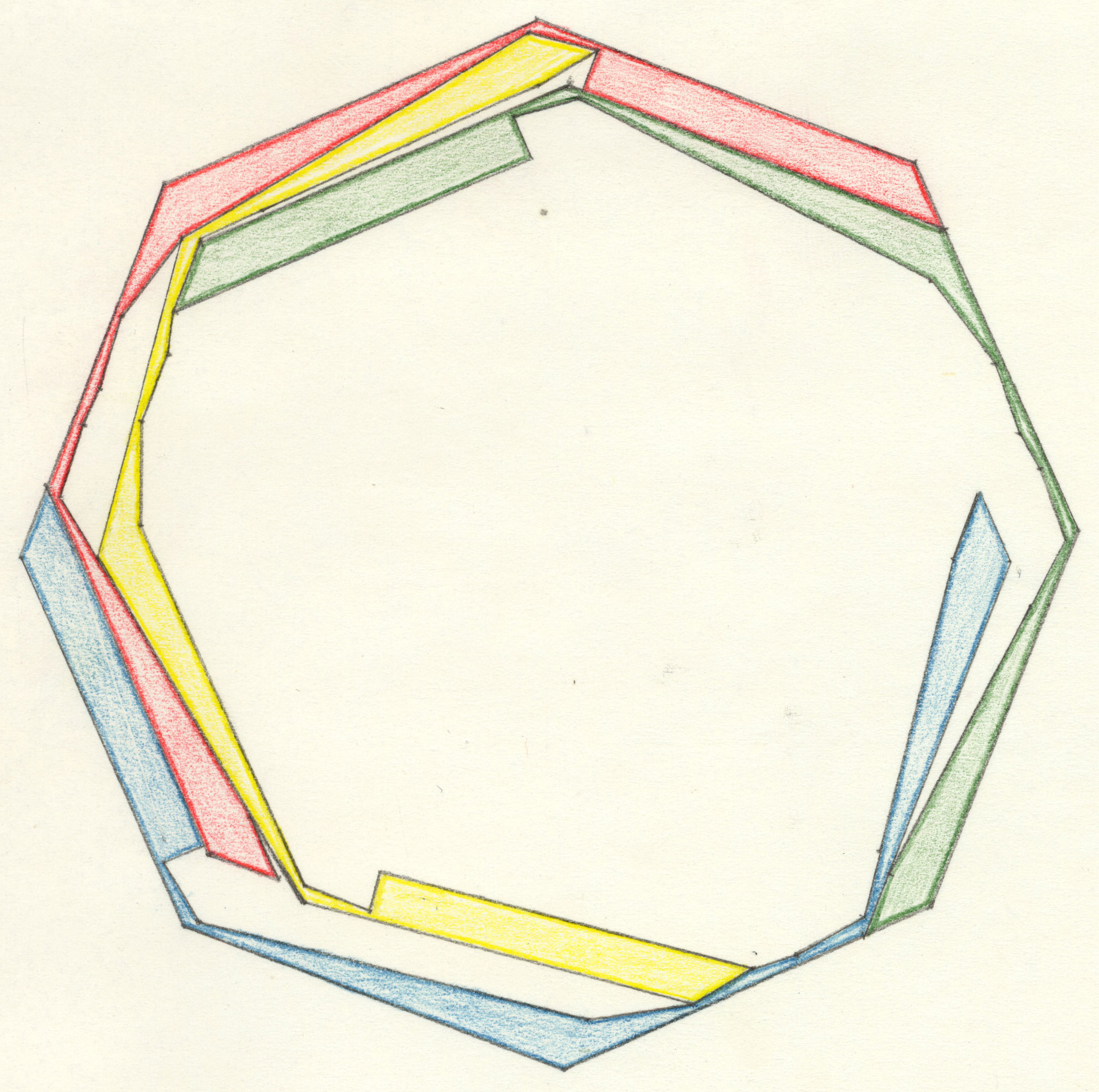n = 9   Nonagon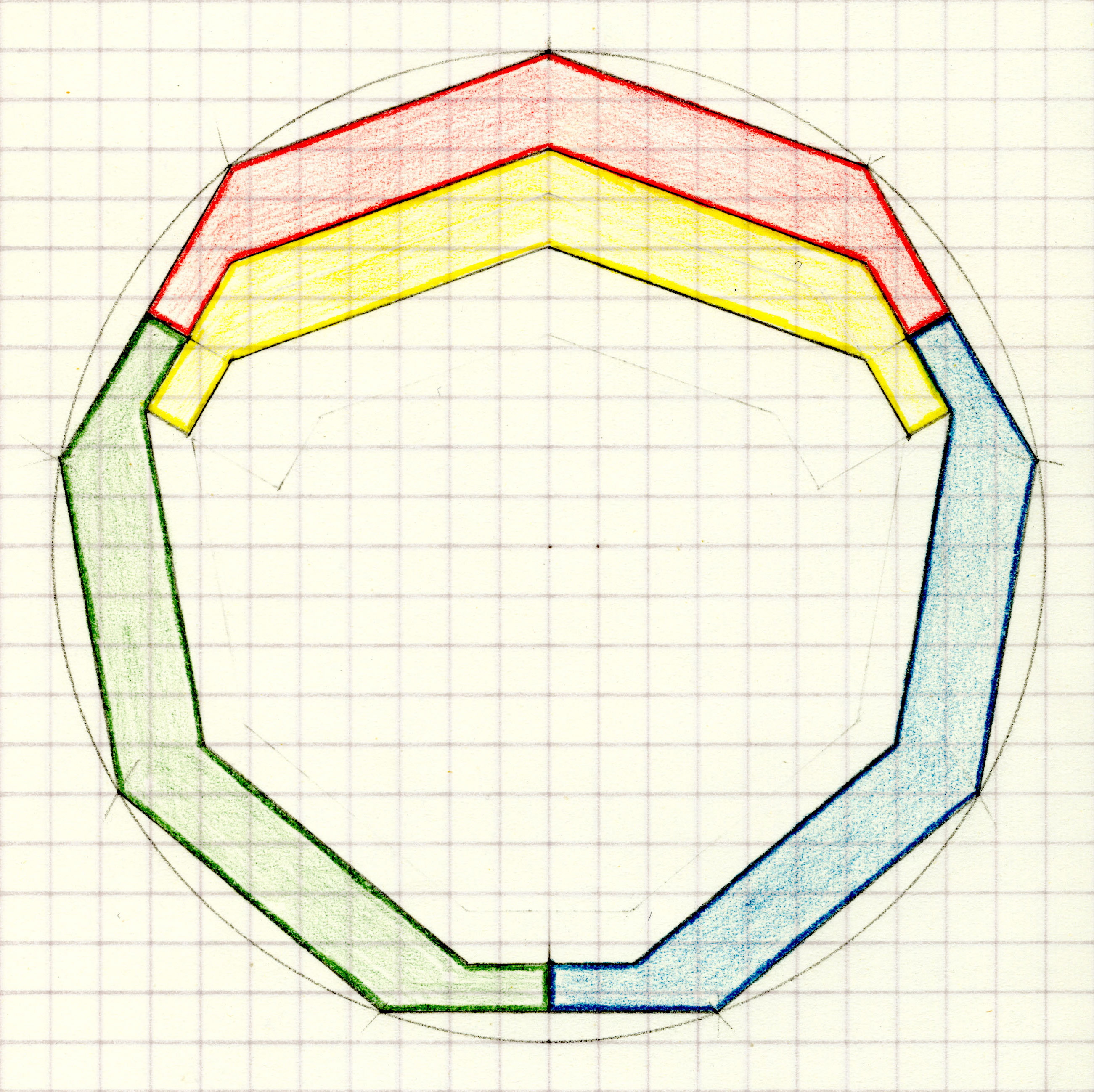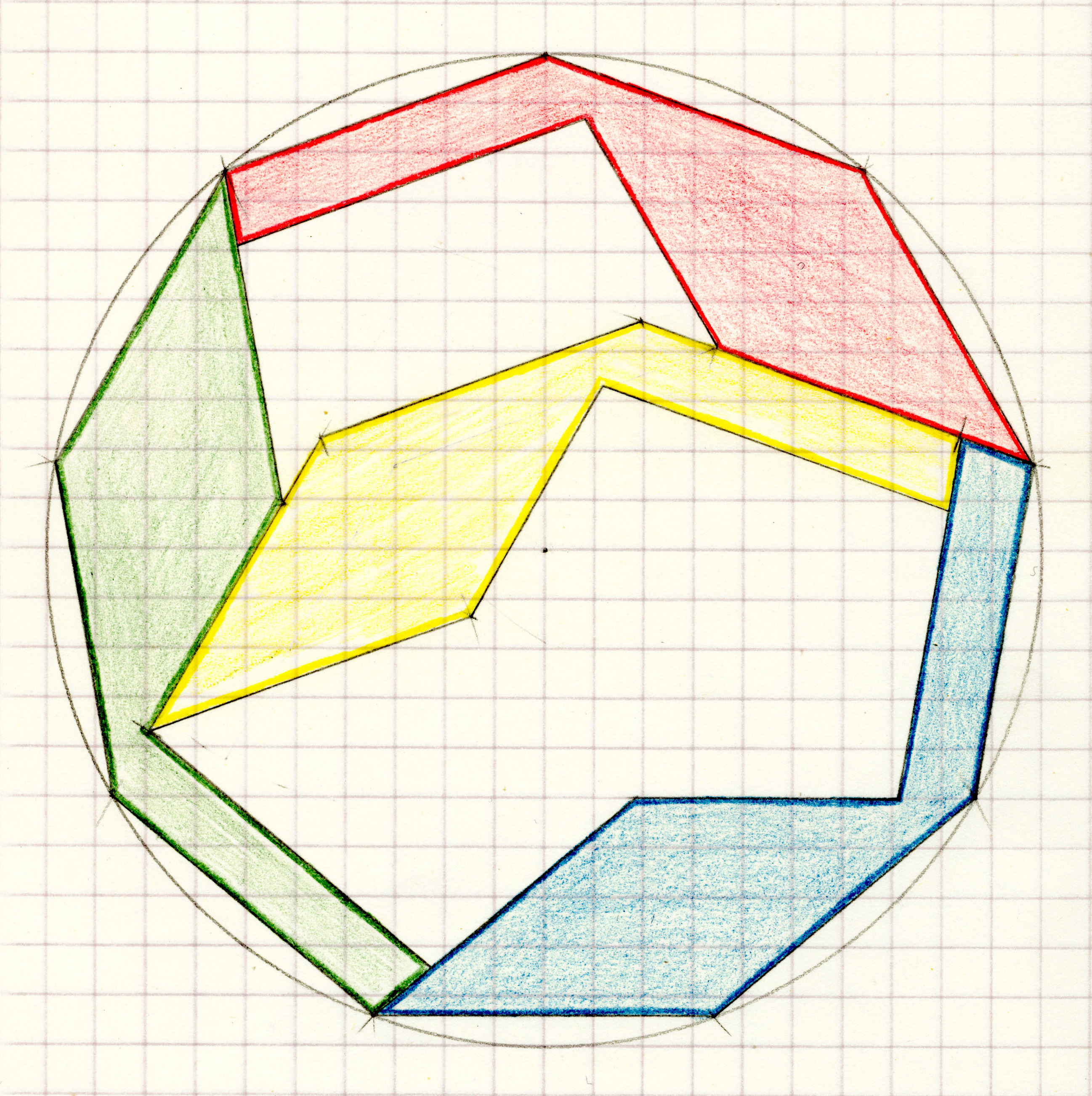n = 10   Decagon     Should be proved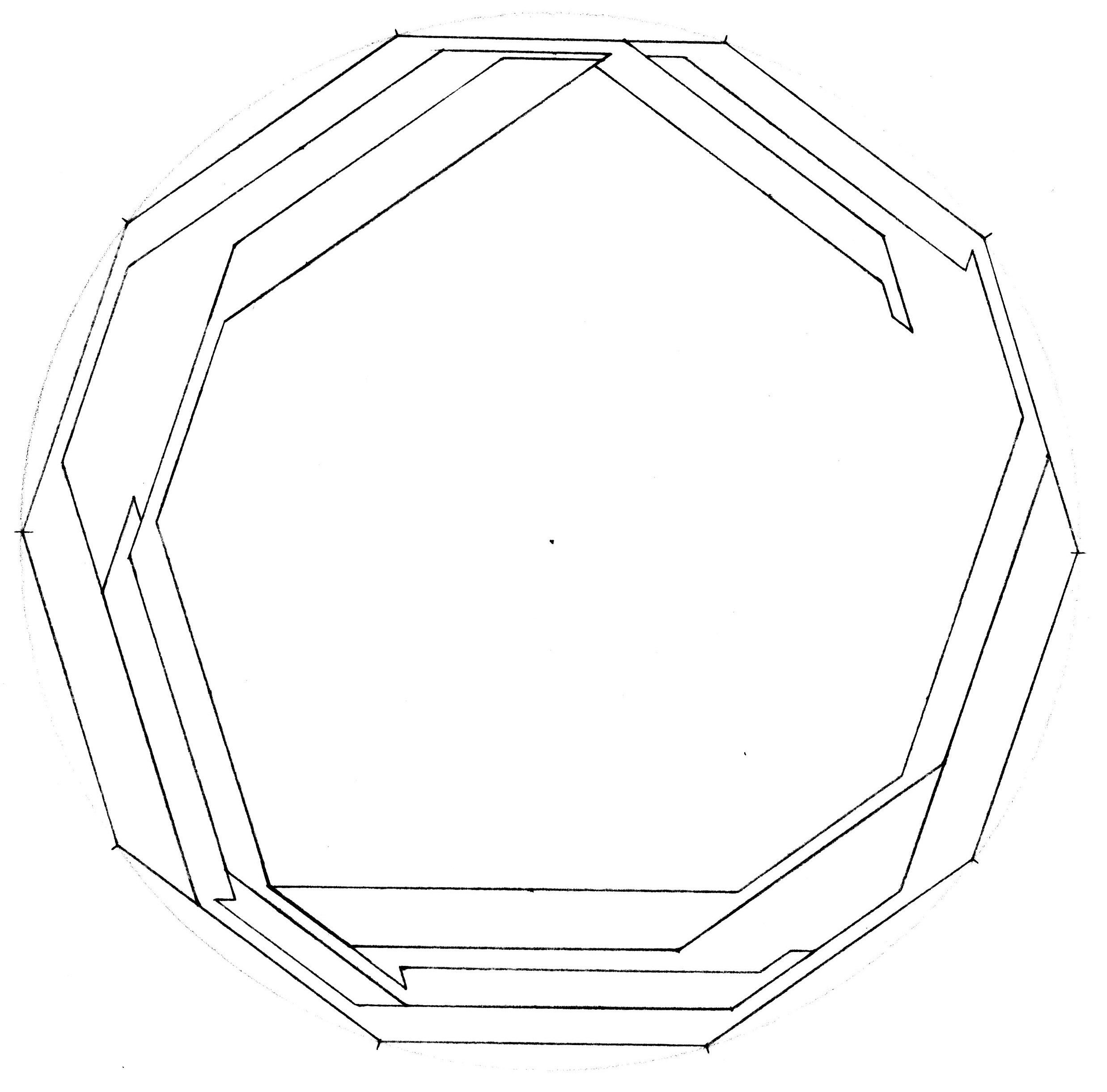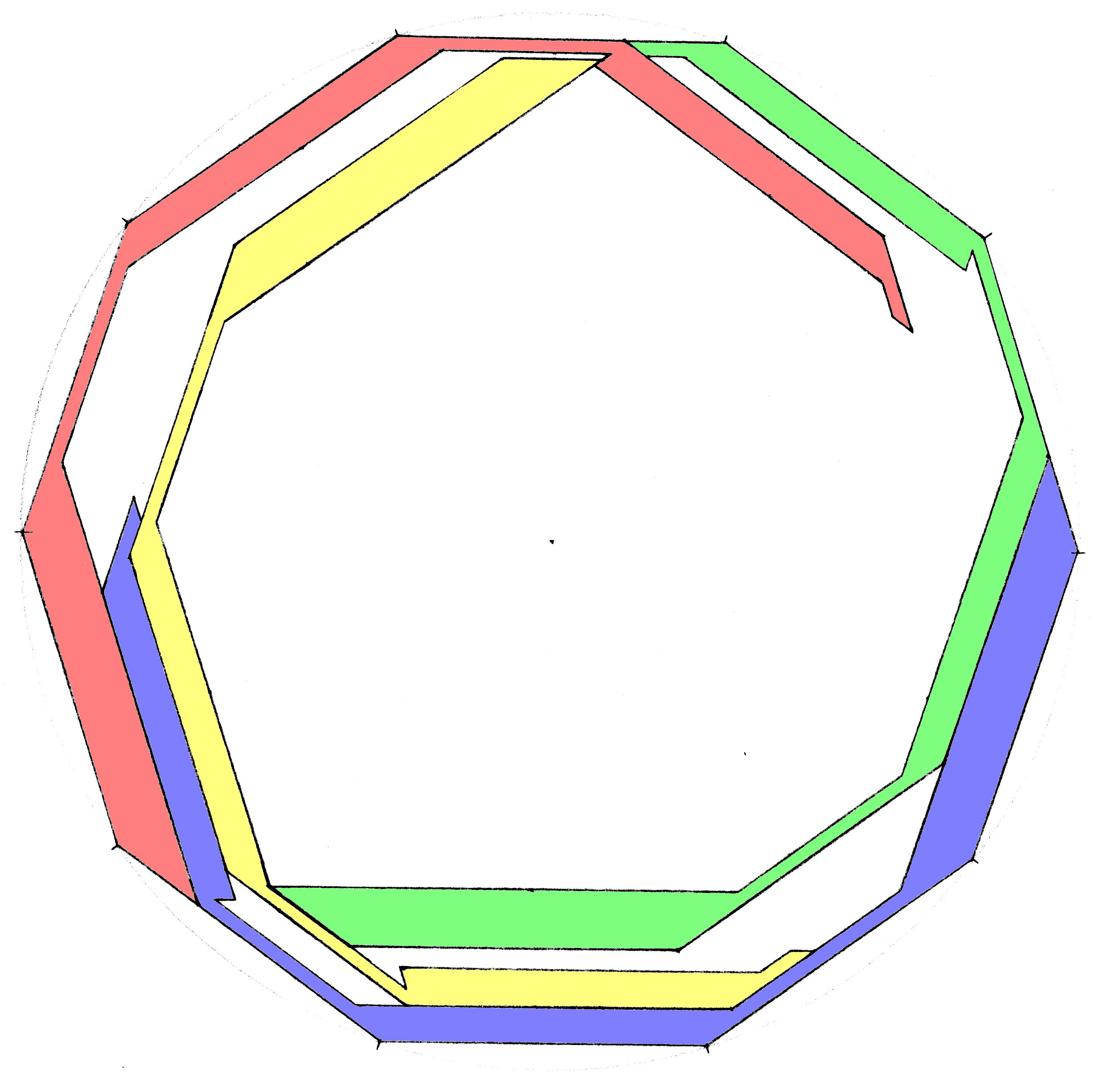n = 11   undecagon     Should be proved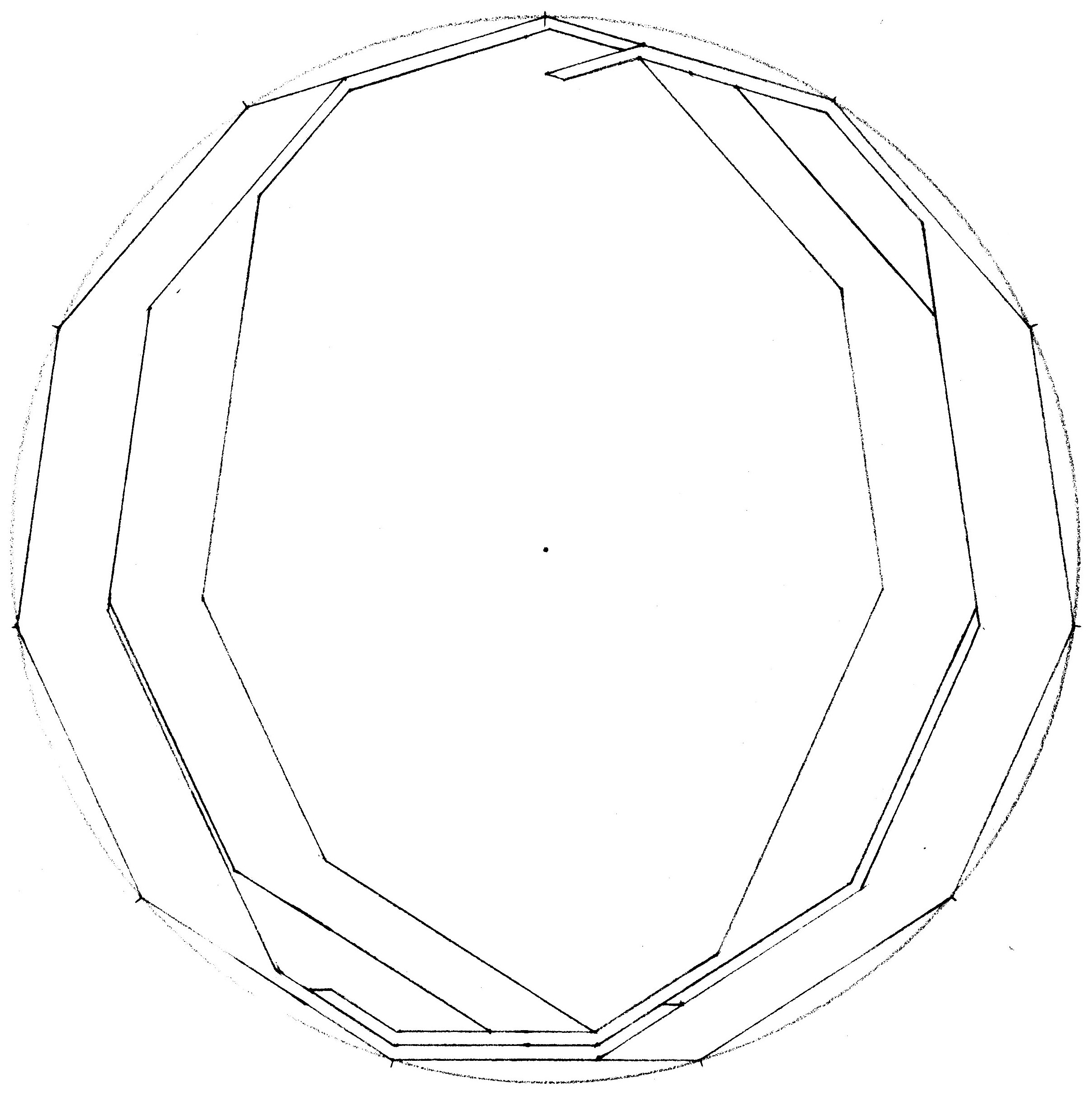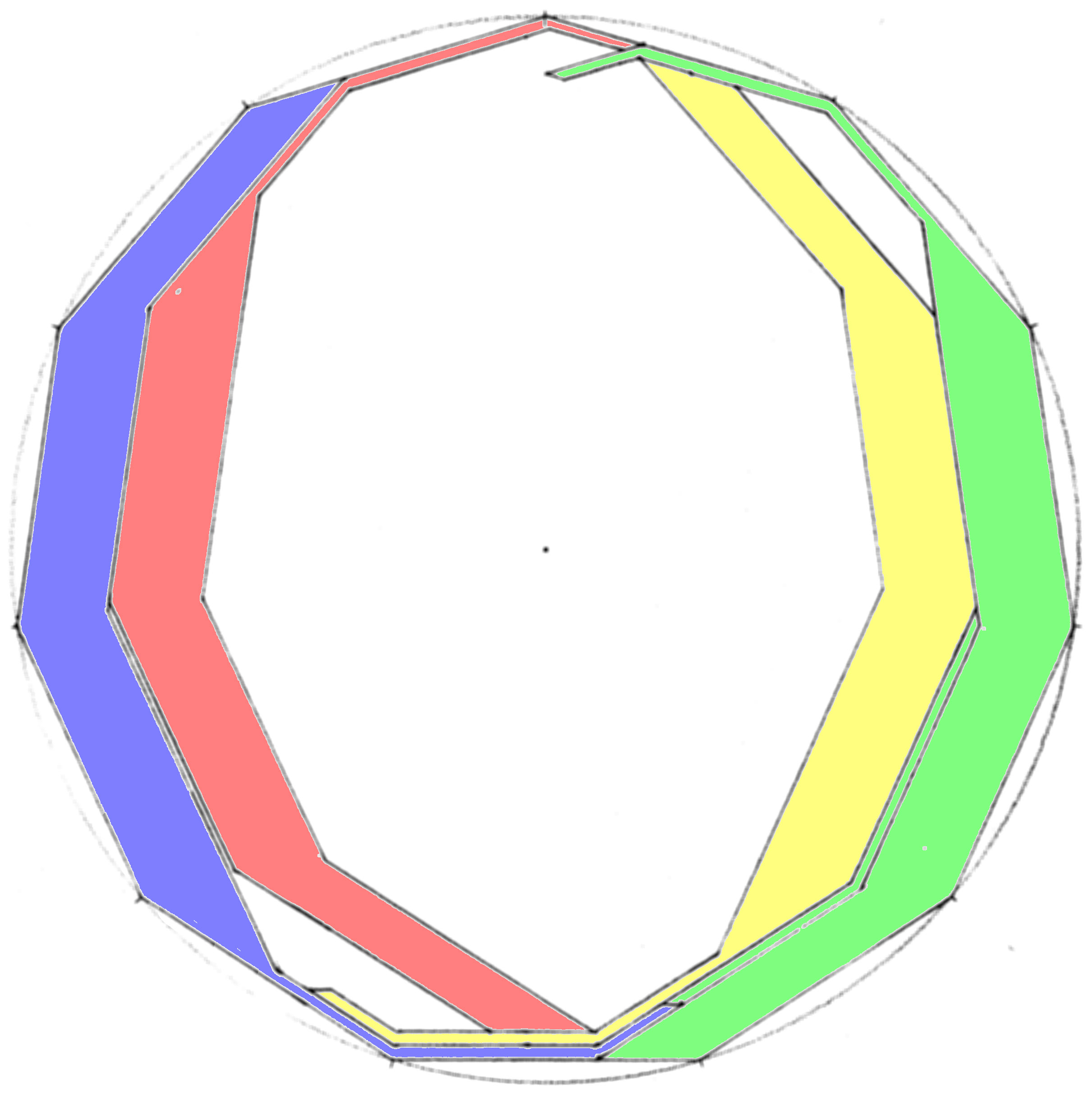n = 12   Dodecagon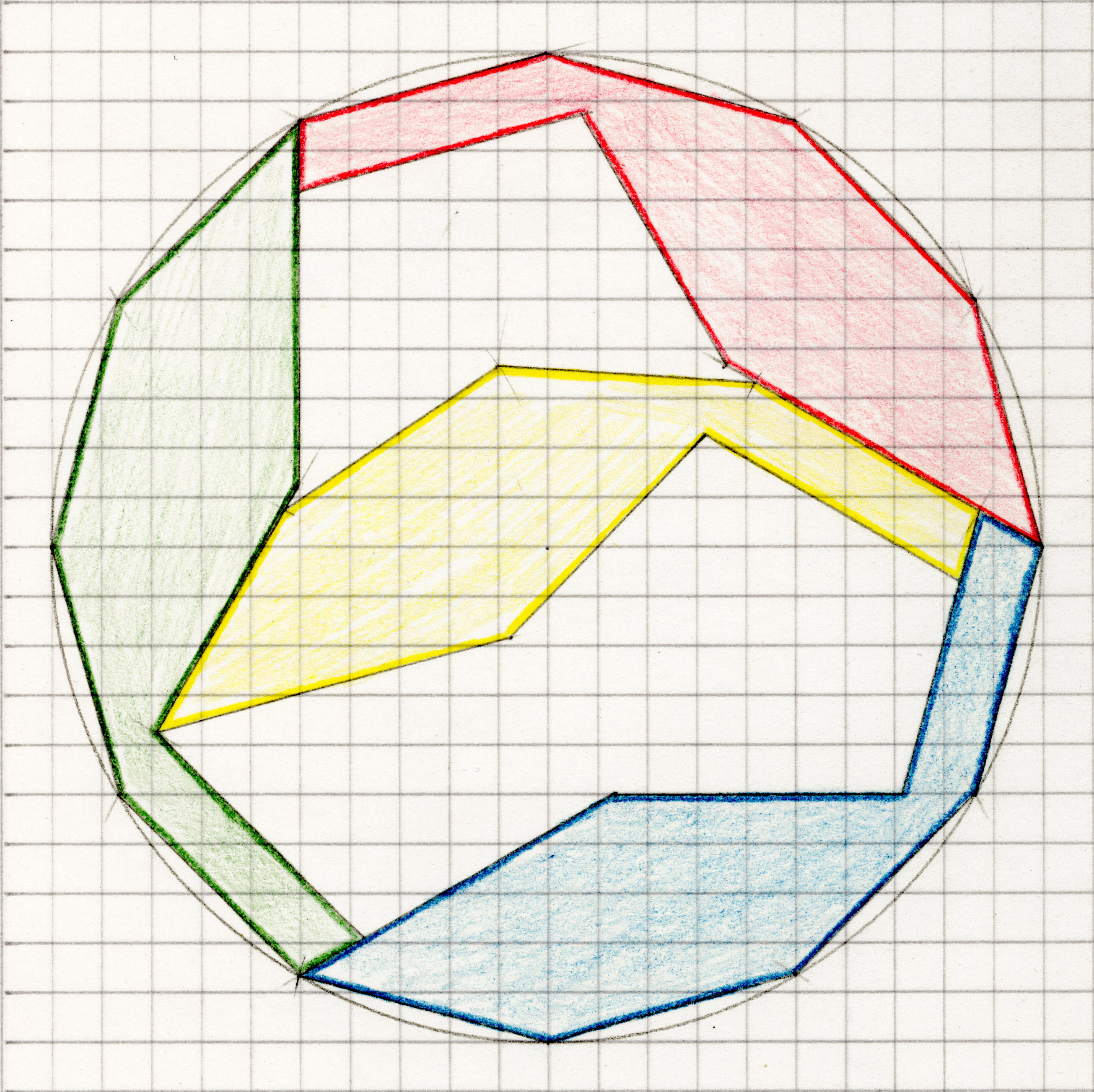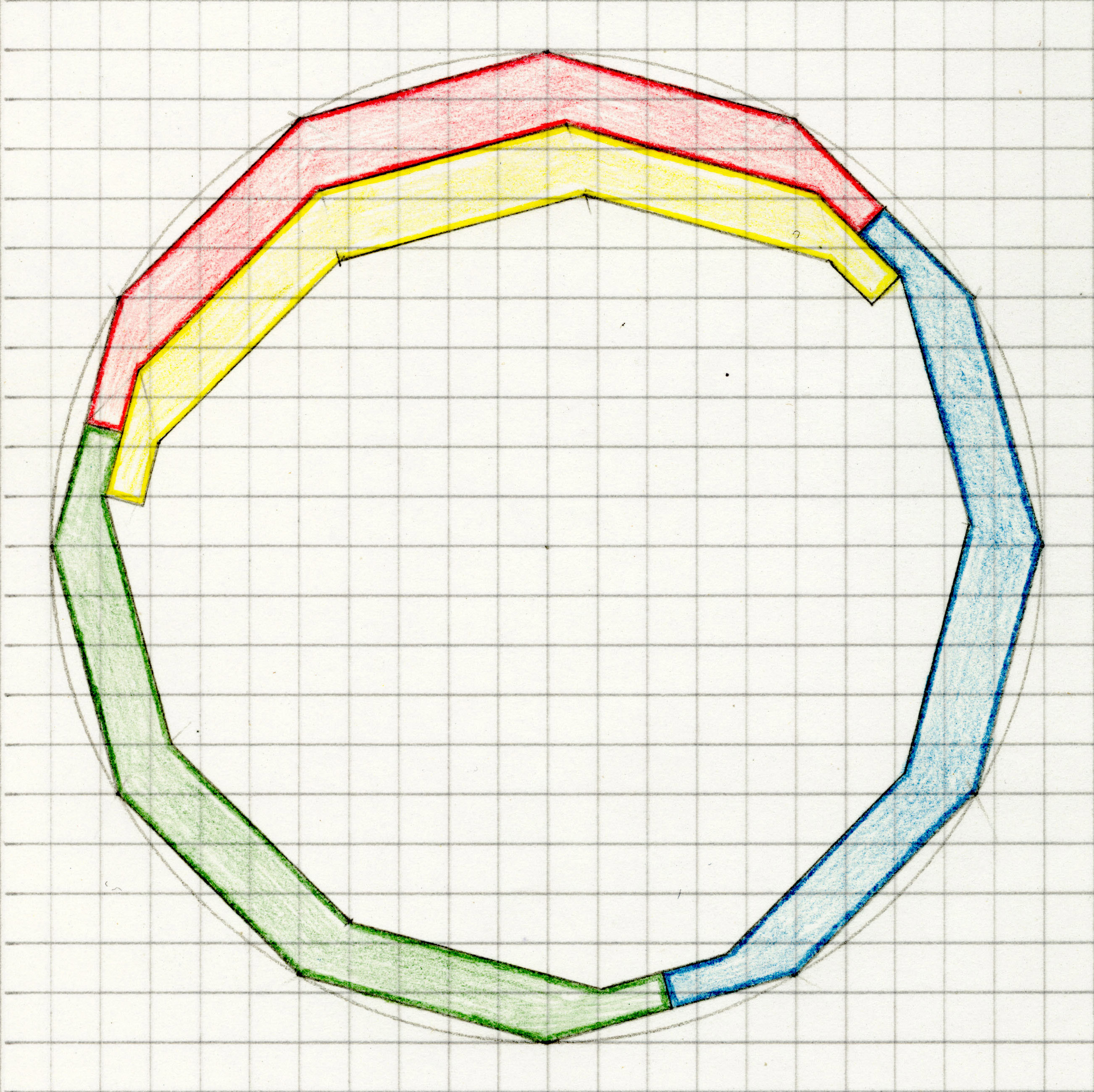Previous Question Tetrads Contents Next Question